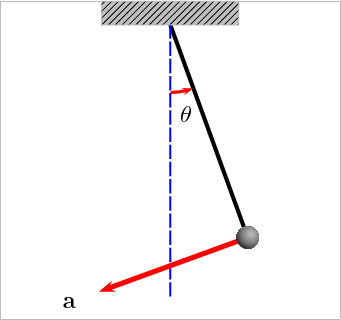## Physical/Compound pendulum Calculator

 I want to calculate Period(T) Center of Mass or Moment of Inertia(I) Mass(M) Acceleration of Gravity(g) Distance from Center of Mass to Pivot(D) Center of Mass or Moment of Inertia(I) = kg-m2 Mass(M) = kg Acceleration of Gravity(g) = m/s2 Distance from Center of Mass to Pivot(D) = m Period(T) = s

The physical pendulum also called compound pendulum is any swinging rigid body free to rotate about a fixed horizontal axis. The animation below is a pendulum.### Physical Pendulum formula

Period: T2 = 4*PI2*I / MgD

where, D = Distance from Center of Mass to Pivot, g = Acceleration of Gravity, M = Mass, I = Center of Mass or Moment of Inertia, T = Period.

For example, when Pi is 3.14, Center of mass or moment of Inertia is 2, Mass is 3, Distance is 4, then T = 0.82S.

Thinkcalculator.com provides you helpful and handy calculator resources.